# Question & Answer: Write a program that reads in the center coordinates and radii of three circles, A, B and C, and reports the location of…..

Write a program that reads in the center coordinates and radii of three circles, A, B and C, and reports the location of a query point relative to the circles. The location can be one of eight possibilities: contained in A, B and C, contained in A and B, contained in A and C, contained in B and C, contained in A, contained in B, contained in C, not contained in any circles.
1. Prompt and read in the x and y coordinates of the center of circle A;
3. Prompt and read in the x and y coordinates of the center of circle B;
5. Prompt and read in the x and y coordinates of the center of circle C;
7. Prompt and read in the x and y coordinates of the query point;
8. Point (x ; y ) is contained in the circle with center (c x ; c y ) and radius r if: q(x   c x )2 + (y   c y )2 r :
By applying this equation to each circle and the query point, determine which circles contain the query point.
Note: The operator ^ is NOT the square operator in C++. To compute (x   c x )2 in C++, create a variable to hold x   c x and the variable with itself. Same for (y   c y )2.
9. Output should be one of the following:
Circles A, B, and C contain point (x,y). Circles A and B contain point (x,y). Circles A and C contain point (x,y). Circles B and C contain point (x,y). Circle A contains point (x,y). Circle B contains point (x,y). Circle C contains point (x,y). No circle contains point (x,y).
In the output x and y should be replaced by the oating point numbers which are the actual coordinates of x and y .
10. Circle center coordinates, query point coordinates and circle radii are oating point numbers. Use double precision.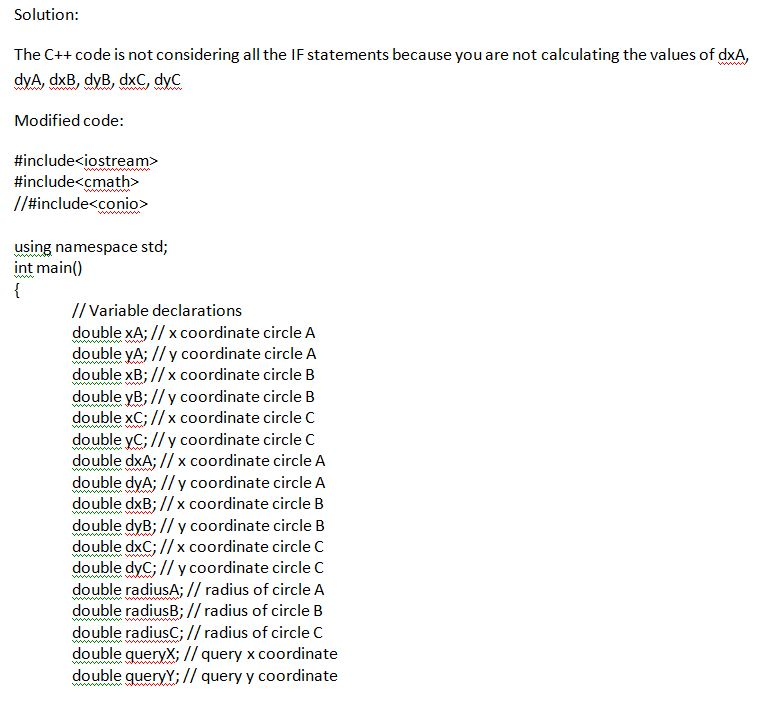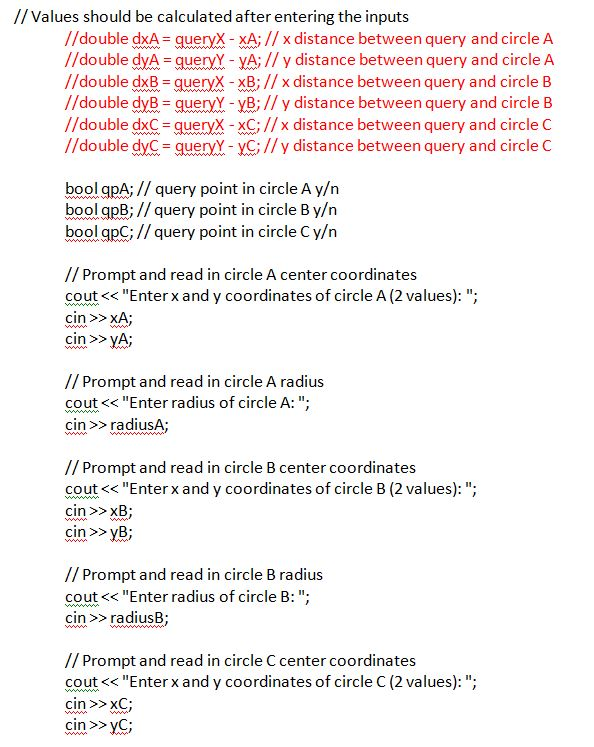Don't use plagiarized sources. Get Your Custom Essay on
Question & Answer: Write a program that reads in the center coordinates and radii of three circles, A, B and C, and reports the location of…..
GET AN ESSAY WRITTEN FOR YOU FROM AS LOW AS \$13/PAGE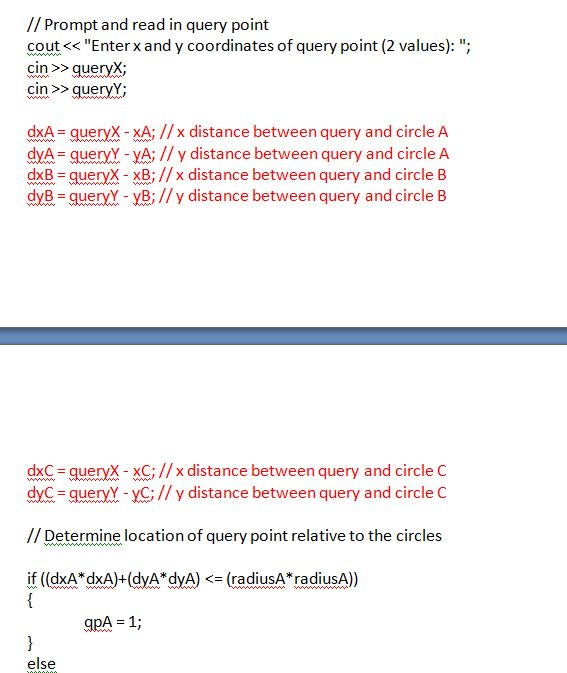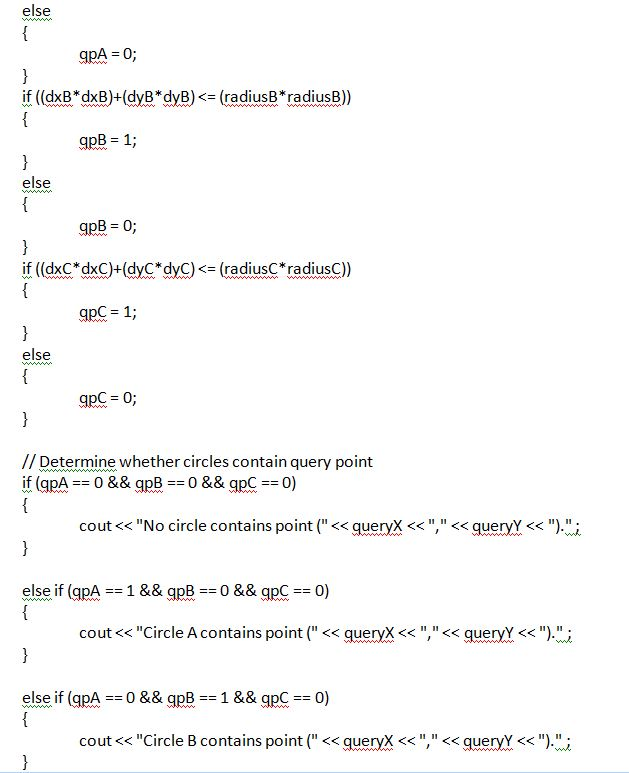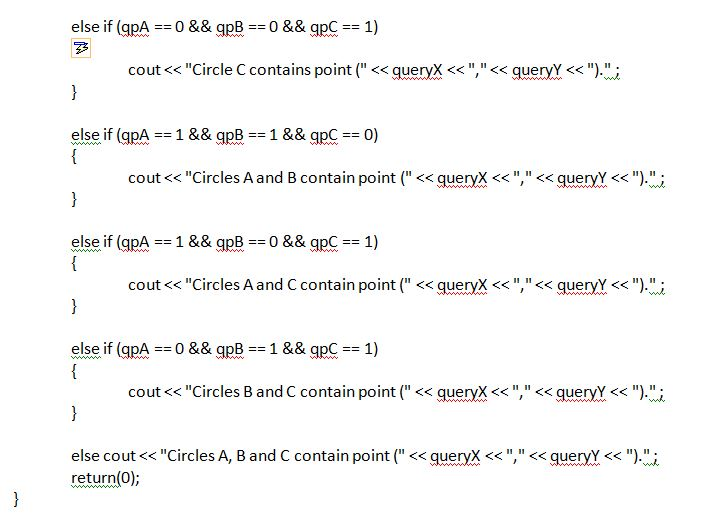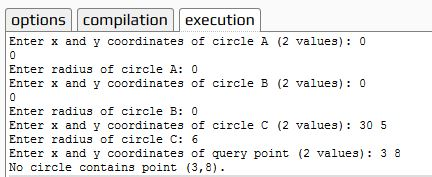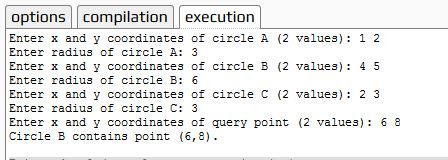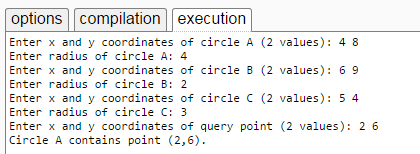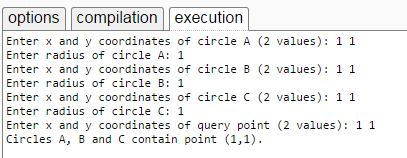Code:

#include<iostream>
#include<cmath>
//#include<conio>

using namespace std;
int main()
{
// Variable declarations
double xA; // x coordinate circle A
double yA; // y coordinate circle A
double xB; // x coordinate circle B
double yB; // y coordinate circle B
double xC; // x coordinate circle C
double yC; // y coordinate circle C
double dxA; // x coordinate circle A
double dyA; // y coordinate circle A
double dxB; // x coordinate circle B
double dyB; // y coordinate circle B
double dxC; // x coordinate circle C
double dyC; // y coordinate circle C
double queryX; // query x coordinate
double queryY; // query y coordinate
//double dxA = queryX – xA; // x distance between query and circle A
//double dyA = queryY – yA; // y distance between query and circle A
//double dxB = queryX – xB; // x distance between query and circle B
//double dyB = queryY – yB; // y distance between query and circle B
//double dxC = queryX – xC; // x distance between query and circle C
//double dyC = queryY – yC; // y distance between query and circle C
bool qpA; // query point in circle A y/n
bool qpB; // query point in circle B y/n
bool qpC; // query point in circle C y/n

// Prompt and read in circle A center coordinates
cout << “Enter x and y coordinates of circle A (2 values): “;
cin >> xA;
cin >> yA;

cout << “Enter radius of circle A: “;

// Prompt and read in circle B center coordinates
cout << “Enter x and y coordinates of circle B (2 values): “;
cin >> xB;
cin >> yB;

cout << “Enter radius of circle B: “;

// Prompt and read in circle C center coordinates
cout << “Enter x and y coordinates of circle C (2 values): “;
cin >> xC;
cin >> yC;

cout << “Enter radius of circle C: “;

// Prompt and read in query point
cout << “Enter x and y coordinates of query point (2 values): “;
cin >> queryX;
cin >> queryY;

dxA = queryX – xA; // x distance between query and circle A
dyA = queryY – yA; // y distance between query and circle A
dxB = queryX – xB; // x distance between query and circle B
dyB = queryY – yB; // y distance between query and circle B
dxC = queryX – xC; // x distance between query and circle C
dyC = queryY – yC; // y distance between query and circle C

// Determine location of query point relative to the circles

{
qpA = 1;
}
else
{
qpA = 0;
}
{
qpB = 1;
}
else
{
qpB = 0;
}
{
qpC = 1;
}
else
{
qpC = 0;
}

// Determine whether circles contain query point
if (qpA == 0 && qpB == 0 && qpC == 0)
{
cout << “No circle contains point (” << queryX << “,” << queryY << “).” ;
}

else if (qpA == 1 && qpB == 0 && qpC == 0)
{
cout << “Circle A contains point (” << queryX << “,” << queryY << “).” ;
}

else if (qpA == 0 && qpB == 1 && qpC == 0)
{
cout << “Circle B contains point (” << queryX << “,” << queryY << “).” ;
}

else if (qpA == 0 && qpB == 0 && qpC == 1)
{
cout << “Circle C contains point (” << queryX << “,” << queryY << “).” ;
}

else if (qpA == 1 && qpB == 1 && qpC == 0)
{
cout << “Circles A and B contain point (” << queryX << “,” << queryY << “).” ;
}

else if (qpA == 1 && qpB == 0 && qpC == 1)
{
cout << “Circles A and C contain point (” << queryX << “,” << queryY << “).” ;
}

else if (qpA == 0 && qpB == 1 && qpC == 1)
{
cout << “Circles B and C contain point (” << queryX << “,” << queryY << “).” ;
}

else cout << “Circles A, B and C contain point (” << queryX << “,” << queryY << “).” ;
return(0);
}